# Introduction to Differential Equations: Types and Examples

A differential equation is an equation that involves derivatives of a function. It describes the rate of change of a quantity. Differential equations can be classified into several types, including ordinary differential equations (ODEs) and partial differential equations (PDEs).

1. Ordinary Differential Equations (ODEs): These involve derivatives with respect to only one independent variable.

Example 1.1: First-order linear ODE

(1)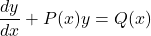Specific example:

(2)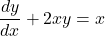Example 1.2: Second-order ODE

(3)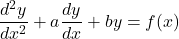Specific example:

(4)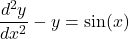2. Partial Differential Equations (PDEs): These involve derivatives with respect to more than one independent variable.

Example 2.1: First-order PDE

(5)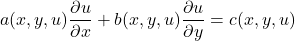Specific example:

(6)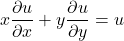Example 2.2: Second-order PDE (The wave equation)

(7)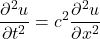Here,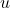represents the amplitude of the wave,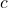is the speed of wave propagation,is time, and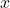is space.

Differential equations are fundamental in expressing the laws and principles governing various natural phenomena. For instance, Newton’s second law, which relates force, mass, and acceleration, can be expressed as a second-order ODE. The heat equation, which describes how temperatures evolve over time within a given region, is a PDE. The Schrödinger equation in quantum mechanics is another example of a PDE.

To solve a differential equation means to find a function (or set of functions) that satisfies the equation. Depending on the nature and complexity of the equation, solutions can be found analytically or may require numerical methods.